### Probability Class 10th Mathematics Tamilnadu Board Solution

##### Question 1.If ϕ is an impossible event, then P(ϕ) =A. 1B.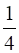C. 0D.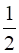Answer:P(ϕ) = 0 since ϕ is an impossible event.Question 2.If S is the sample space of a random experiment, then P(S) =A. 0B.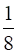C.D. 1Answer:Since S is the sample space thus P(S) = 1Question 3.If p is the probability of an event A, then p satisfiesA. 0 < p < 1B. 0 ≤ p ≤ 1C. 0 ≤ p < 1D. 0 < p ≤ 1Answer:Probability always lies between 0 and 1.Hence, P(A) = p⇒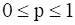Question 4.Let A and B be any two events and S be the corresponding sample space.Then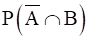=A. P(B) – P(A ∩ B)B. P(A ∩ B) – P(B)C. P (S)D. P [(A ∪ B)’]Answer:=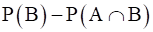Asimplies the portion of only event B. hence event A and the intersection between these two are not counted.Question 5.The probability that a student will score centum in mathematics is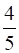. The probability that he will not score centum isA.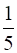B.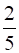C.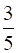D.Answer:The probability that a student will score centum in mathematics is.Thus probability that he will not score centum is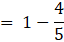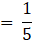Question 6.If A and B are two events such thatP (A) = 0.25, P (B) = 0.05 and P(A ∩ B) = 0.14, then P(A ∪ B) =A. 0.61B. 0.16C. 0.14D. 0.6Answer: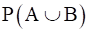= P (A)+ P (B)-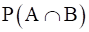⇒= 0.25+0.05-0.14 = 0.16Question 7.There are 6 defective items in a sample of 20 items. One item is drawn at random. The probability that it is a non-defective item isA.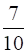B. 0C.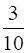D.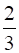Answer:No of defective items = 6Total no of samples = 20Probability that the item is not defective = 1-6/20=Question 8.If A and B are mutually exclusive events and S is the sample space such that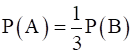and S = A ∪ B, then P (A) =A.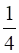B.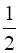C.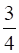D.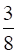Answer:Since the event are mutually exclusive the intersection is 0.Thus P(AUB) = P(A)+P(B)⇒ P(AUB) = P(A)+3.P(A)⇒ 1 = 4.P(A)P(A) =Question 9.The probabilities of three mutually exclusive events A, B and C are given by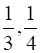and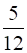. Then P(A ∪ B ∪ C) isA.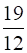B.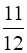C.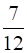D. 1Answer:Since the events are mutually exclusive the intersections between the respective events will be 0.P(AUBUC) = P(A)+P(B)+P(C)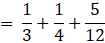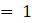Question 10.If P(A) = 0.25, P (B) = 0.50, P(A ∩ B) = 0.14 then P (neither A nor B) =A. 0.39B. 0.25C. 0.11D. 0.24Answer:P(AUB) = 0.25-0.50-0.14 = 0.61Thus P (neither A nor B) = 1-P(AUB) = 1-0.61 = 0.39Question 11.A bag contains 5 black balls, 4 white balls and 3 red balls. If a ball is selected at random, the probability that it is not red isA.B.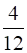C.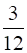D.Answer:Total no of balls = 12No of black balls = 5No of white balls = 4No of red balls = 3Probability thst the ball is not red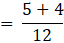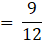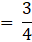Question 12.Two dice are thrown simultaneously. The probability of getting a doublet isA.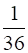B.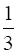C.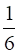D.Answer:The outcomes on throwing a dice twice are: (1,1),(1,2),(1,3),(1,4),(1,5),(1,6)(2,1),(2,2),(2,3),(2,4),(2,5),(2,6)(3,1),(3,2),(3,3),(3,4),(3,5),(3,6)(4,1),(4,2),(4,3),(4,4),(4,5),(4,6)(5,1),(5,2),(5,3),(5,4),(5,5),(5,6)(61),(6,2),(63),(6,4),(6,6),(6,6)Total outcomes = 36Probable outcome of a duplet = 6⇒ probability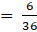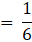Question 13.A fair die is thrown once. The probability of getting a prime or composite number isA. 1B. 0C.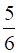D.Answer:Total outcomes = 6Thee are 5 numbers which satisfy the prime and composite: 2,3,4,5,6Thus probability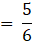Question 14.Probability of getting 3 heads or 3 tails in tossing a coin 3 times isA.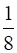B.C.D.Answer:The outcomes when three coins are tossed aare:(H H H) (H H T) (H T H) (T H H) (T T H) (T H T) (H T T) (T T T)No of probable outcomes of getting of 3 heada or 3 tails = 2⇒ probability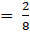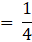Question 15.A card is drawn from a pack of 52 cards at random. The probability of getting neither an ace nor a king card isA.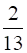B.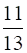C.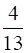D.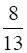Answer:No of ace and king cards = 4+4 = 8Total no of cards = 52Thus no of cards neither ace nor king = 52-8 = 44Probability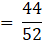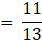Question 16.The probability that a leap year will have 53 Fridays or 53 Saturdays isA.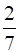B.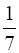C.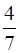D.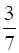Answer:Leap year has 52 weeks and 2 more days. For 53 Fridays those two should be Thursday, Friday or Friday, Saturday. Hence probability of 53 FridaysSimilarly probability of 53 Saturdays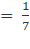Let these two events be A and B respectively.Now A and B can't occur simultaneously as there can't be any pair consisting of Friday and Saturday.So P(A or B) = P(A)+P(B)-P(AB) =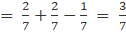Question 17.The probability that a non-leap year will have 53 Sundays and 53 Mondays isA.B.C.D. 0Answer:Since it is a non leap year thus there cannot be 53 number of Sundays and Mondays each. Thus its probability is 0.Question 18.The probability of selecting a queen of hearts when a card is drawn from a pack of 52 playing cards isA.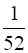B.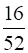C.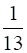D.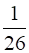Answer:There are 13 hearts of which 1 is queen of hearts out of 52.probability of selecting a queen of hearts when a card is drawn from a pack of 52 playing cards is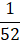Question 19.Probability of sure event isA. 1B. 0C. 100D. 0.1Answer:Since the event is sure, the probability of a sure event = 1.Question 20.The outcome of a random experiment results in either success or failure. If the probability of success is twice the probability of failure, then the probability of success isA.B.C. 1D. 0Answer:Let the probability of failure be x, then the probability of success is 2x.Thus probability of success =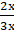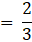PDF FILE TO YOUR EMAIL IMMEDIATELY PURCHASE NOTES & PAPER SOLUTION. @ Rs. 50/- each (GST extra)

HINDI ENTIRE PAPER SOLUTION

MARATHI PAPER SOLUTION

SSC MATHS I PAPER SOLUTION

SSC MATHS II PAPER SOLUTION

SSC SCIENCE I PAPER SOLUTION

SSC SCIENCE II PAPER SOLUTION

SSC ENGLISH PAPER SOLUTION

SSC & HSC ENGLISH WRITING SKILL

HSC ACCOUNTS NOTES

HSC OCM NOTES

HSC ECONOMICS NOTES

HSC SECRETARIAL PRACTICE NOTES

2019 Board Paper Solution

HSC ENGLISH SET A 2019 21st February, 2019

HSC ENGLISH SET B 2019 21st February, 2019

HSC ENGLISH SET C 2019 21st February, 2019

HSC ENGLISH SET D 2019 21st February, 2019

SECRETARIAL PRACTICE (S.P) 2019 25th February, 2019

HSC XII PHYSICS 2019 25th February, 2019

CHEMISTRY XII HSC SOLUTION 27th, February, 2019

OCM PAPER SOLUTION 2019 27th, February, 2019

HSC MATHS PAPER SOLUTION COMMERCE, 2nd March, 2019

HSC MATHS PAPER SOLUTION SCIENCE 2nd, March, 2019

SSC ENGLISH STD 10 5TH MARCH, 2019.

HSC XII ACCOUNTS 2019 6th March, 2019

HSC XII BIOLOGY 2019 6TH March, 2019

HSC XII ECONOMICS 9Th March 2019

SSC Maths I March 2019 Solution 10th Standard11th, March, 2019

SSC MATHS II MARCH 2019 SOLUTION 10TH STD.13th March, 2019

SSC SCIENCE I MARCH 2019 SOLUTION 10TH STD. 15th March, 2019.

SSC SCIENCE II MARCH 2019 SOLUTION 10TH STD. 18th March, 2019.

SSC SOCIAL SCIENCE I MARCH 2019 SOLUTION20th March, 2019

SSC SOCIAL SCIENCE II MARCH 2019 SOLUTION, 22nd March, 2019

XII CBSE - BOARD - MARCH - 2019 ENGLISH - QP + SOLUTIONS, 2nd March, 2019

HSC Maharashtra Board Papers 2020

(Std 12th English Medium)

HSC ECONOMICS MARCH 2020

HSC OCM MARCH 2020

HSC ACCOUNTS MARCH 2020

HSC S.P. MARCH 2020

HSC ENGLISH MARCH 2020

HSC HINDI MARCH 2020

HSC MARATHI MARCH 2020

HSC MATHS MARCH 2020

SSC Maharashtra Board Papers 2020

(Std 10th English Medium)

English MARCH 2020

HindI MARCH 2020

Hindi (Composite) MARCH 2020

Marathi MARCH 2020

Mathematics (Paper 1) MARCH 2020

Mathematics (Paper 2) MARCH 2020

Sanskrit MARCH 2020

Sanskrit (Composite) MARCH 2020

Science (Paper 1) MARCH 2020

Science (Paper 2)

MUST REMEMBER THINGS on the day of Exam

Are you prepared? for English Grammar in Board Exam.

Paper Presentation In Board Exam

How to Score Good Marks in SSC Board Exams

Tips To Score More Than 90% Marks In 12th Board Exam

How to write English exams?

How to prepare for board exam when less time is left

How to memorise what you learn for board exam

No. 1 Simple Hack, you can try out, in preparing for Board Exam

How to Study for CBSE Class 10 Board Exams Subject Wise Tips?

JEE Main 2020 Registration Process – Exam Pattern & Important Dates

NEET UG 2020 Registration Process Exam Pattern & Important Dates

How can One Prepare for two Competitive Exams at the same time?

8 Proven Tips to Handle Anxiety before Exams!